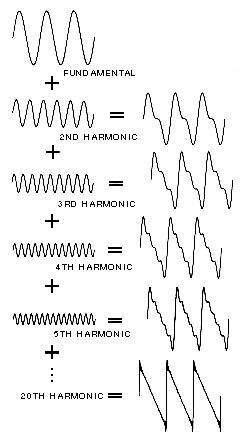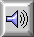FOURIER SYNTHESIS

The construction of a PERIODIC signal on the basis of Fourier coefficients which give the AMPLITUDE and PHASE angle of each component sine wave HARMONIC. These coefficients are obtained through FOURIER ANALYSIS. The synthesis technique is also called additive synthesis.

As supported by the LAW OF SUPERPOSITION, sine tones of varying amplitudes will combine to form a complex harmonic SPECTRUM. If every period of sound has been subjected to Fourier analysis by a computer, then this information can be used to reproduce a sound indistinguishable from the original by means of Fourier synthesis. However, onset TRANSIENTs being APERIODIC are difficult to reproduce accurately. The more general form of transferring the frequency domain to the time domain is termed the inverse Fourier transform.Successive approximations of a sawtooth wave by addition of harmonics with amplitude inversely proportional to the harmonic number. The resultant waveform at each stage of addition is shown at right.Sound Example: Addition of the first 14 sine wave harmonics resulting in the successive approximation of a sawtooth wave.

home